﻿ Relativistic quantum field theory is wrong.

# Quantum field theory is useless.

## How was quantum field theory born ?

### [ Quantum mechanics + special relativity = quantum field theory. ]

(Fig.1)  Quantum field theory lacks reality.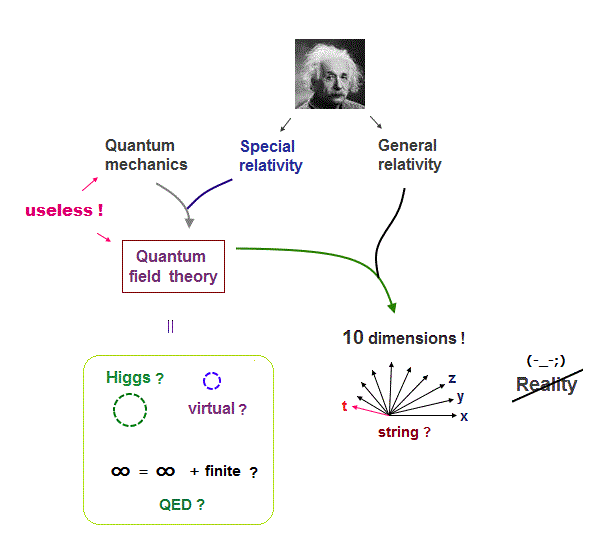When you want to know what the photon actually is, the present physics forces you to learn quantum field theory.

What the heck is this quantum field theory ?
In fact, this quantum field theory is just a collection of meaningless symbols.

Quantum field theory was made by combining quantum mechanics and Einstein relativity.

Quantum electrodynamics (= QED ) using unreal virtual particles and Higgs also belong to this category.

The only theory of everyhing 10 spacetime dimensional string ( or M ) theory was gotten, adding general relativity to it.

Unfortunately, all these quantum field theories lack reality, and useless for us.

## Einstein mc2 is the basis of quantum field theory.

### [ Einstein mc2 produced Higgs, quarks, virtual particles. ]

(Fig.2)  ↓ Quantum field theory governs all particles.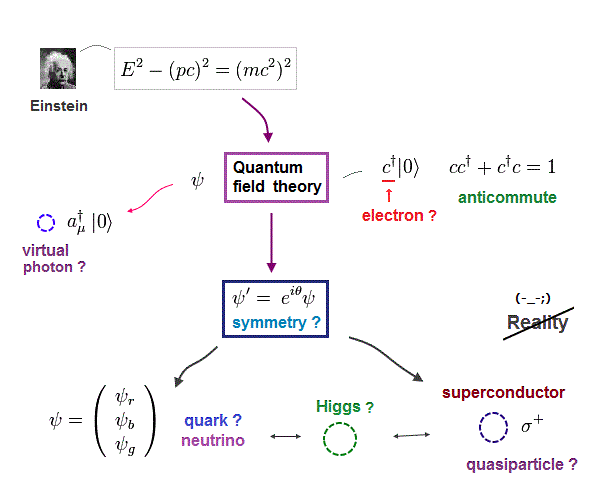Though the media likes to mislead people using showy images about particle physics, these images are all fake.

Ordinary people don't know the fact that abstract quantum filed theory governs all particles such as electron, quark and neutrino ..

Einstein relation of mc2 is applied to this relativistic Dirac equation, which is the basis of unreal QED, Higgs and forces.

All these quantum field theories have No ability to show concrete atomic pictures, so they lack reality.

In fact, they hide true paradox of Einstein relativity, which destroys all student's careers now !

## How did they derive Einstein famous mc2 ?

### [ Energy and momentum by Einstein relativity. ]

(Fig.3)  Stationary observer (= K ) sees a stationary truck.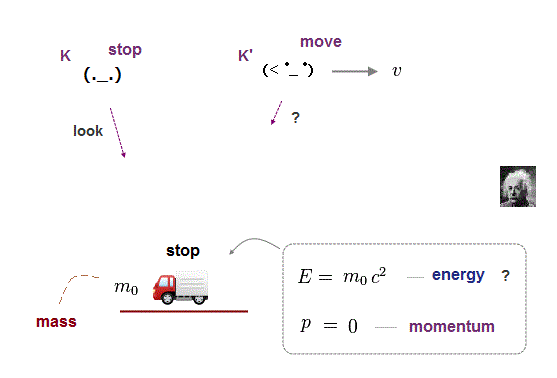Einstein derived famous equation which governs the energy (= E ) and momentum (= p ) of each particle.

Suppose a stationary observer (= K ) sees a stationary truck with a mass m0.
Einstein claimed even this truck at rest has large energy E = m0c2.

The proof of this rest mass energy depends on "antiparticle".
But this elusive antiparticle can be replaced by electron capture in PET.

Furthermore, the antiparticle violates basic physical rule of momentum conservation.

The point is antiparticle cannot be generated only from the "light" in the reverse process of annihilation.  It must rely on collision with other metals, so doubtful.

This Einstein's hypothesis of mc2 lacks direct physical proofs.

## Truck looks heavier seen from moving observer ?

### [ Mass of a truck looks bigger, seen from moving observer ? ]

(Fig.4)  Energy (= E ) and momentum (= p ) of a truck seen from K'.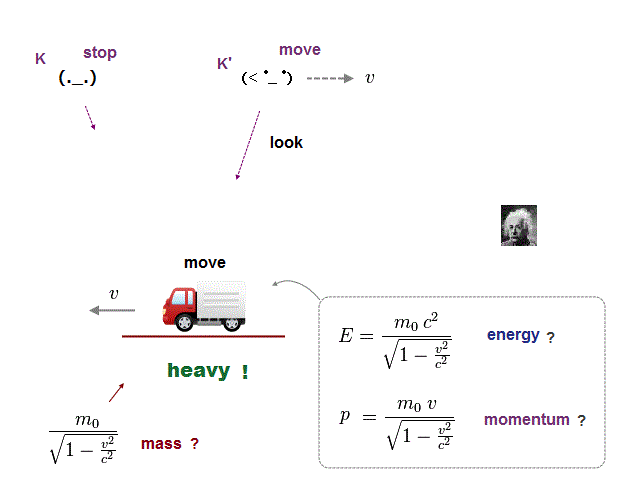Einstein relativity is based on "relative ( not absolute ) motion".
An observer (= K' ) moving at a speed v sees the stationary truck in Fig.4.

Seen from this moving observer, a stationary truck appears to be moving in the opposite direction at v.

Einstein claims the mass of this truck looks heavier, when it appears to be moving.

So the energy (= E ) and momentum (= p ) of the stationary truck become this, seen from moving observer K'.

The point is these are just "Einstein artificial definition" which has nothing to do with the original Lorentz transformation.

## Einstein energy momentum relation.

### [ Energy-momentum relation is invariant in any observer ? ]

(Fig.5)  Relativistic momentum (= p ), energy (= E ).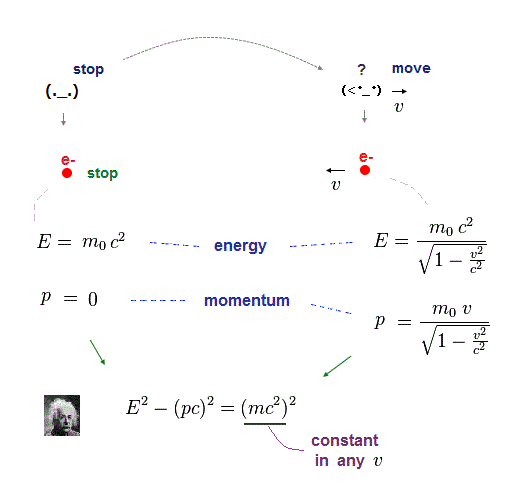From this and this, we can get Einstein's most important relation using relativistic energy (= E ) and momentum (= p ).

The point is the right hand side of Fig.5 always becomes constant =(mc2)2 regardless of any relative velocities "v".

This Einstein important relation is the basis of all quantum field theories such as QED, Higgs, and string theory.

These artificial energy and momentum transform like ( t, x, y, z ) under Lorentz transformation ( see this p.2 )

## Einstein relation → Dirac equation with spin ?

### [ Dirac equation forms the basis of quantum field theory. ]

(Fig.6)  ↓ Linear Dirac equation contains spin ?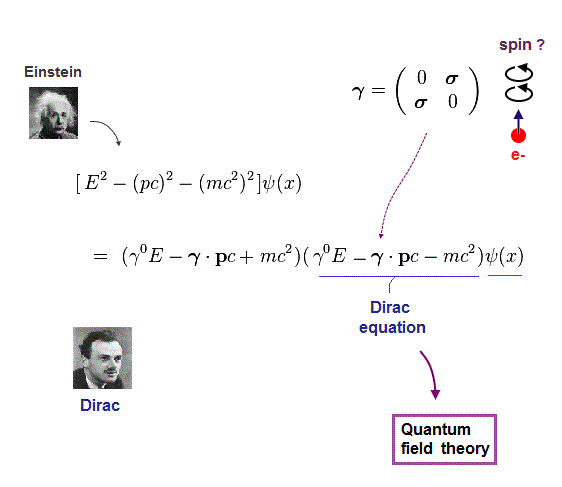Dirac equation is the most important one which forms the basis of all quantum field theories.  The problem is its form is very unrealistic.

Relativistic Dirac equation was gotten by dividing Einstein quadratic relation into linear functions.

In compensation for linear function, Dirac equation must contain 4 × 4 gamma (= γ ) matrices, which consist of spin Pauli matrices (= σ ).

This is the reason they claim Dirac equation succeeded in combining "spin" and relativity.  But "σ = spin ?" is just artificial definition with No grounds.

## Einstein relation includes fatal paradox.

### [ So all quantum field theories, Dirac equation are wrong. ]

(Fig.7)  ↓ Relativistic Dirac equation governs all particles.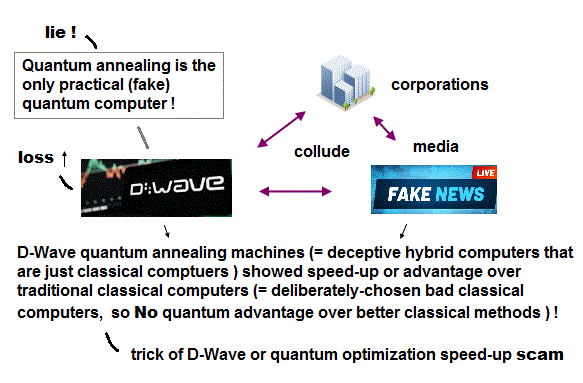The problem is this Einstein important relation between energy and momentum includes fatal paradoxes.

It means all quantum field theories based on Dirac equation are wrong.
This Einstein momentum is inconsistent with de Broglie wave of electron.

Another fatal paradox is related to force.
These paradoxes are hidden by the media and universities from people !

Lorentz transformation itself includes true paradox.
To solve these all paradoxes, we have to admit some medium with the earth.

The faster the particle is moving, the stronger the resistance around the particles becomes.

It is the reason why it's harder to accelerate the faster particle (= looks heavier ? )  The maxium speed of electric field accelerating particle is "c".

## de Broglie wave of a moving electron.

### [ de Broglie wavelength is related to electron's velocity. ]

(Fig.8)  Interference pattern of a single electron is seen.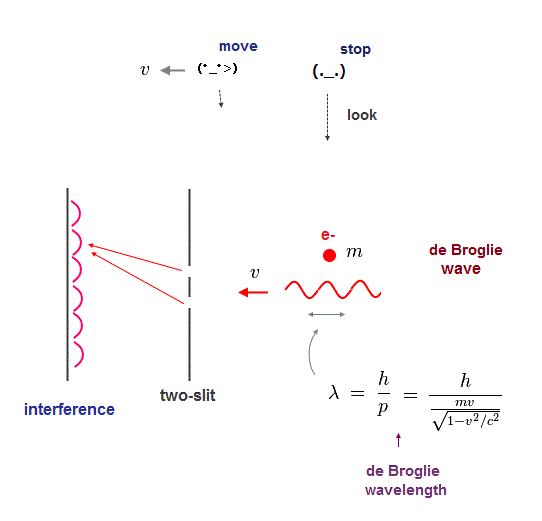Electron's de Broglie wave relation was confirmed in a lot of experiments using double-slits.  de Broglie wavelength λ = h/mv,  v is electron's velocity.

Electron's de Broglie wavelength using relativistic momentum (= p ) is this.

A stationary observer watches the electron moving at a speed "v".
This electron generates its de Broglie wave and causes interference on the screen.

## de Broglie wave vanishes seen from moving observer ?

### [ Seen from an observer moving with an electron, interference vanishes ? ]

(Fig.9)  ↓ Seen from an observer moving with an electron,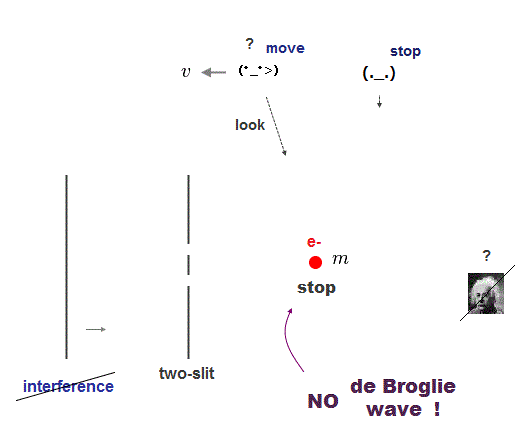Another observer is moving at the same velosity as the electron.
So seen from this moving observer, the electron looks stationary.

This "stationary-looking" electron causes No de Broglie wave, and No interference pattern is seen on the screen !  This is clearly a paradox.

## Interference is seen or unseen !?

### [ Different observers see different interference patterns ? ]

(Fig.10)  de Broglie wave appears or disappears ?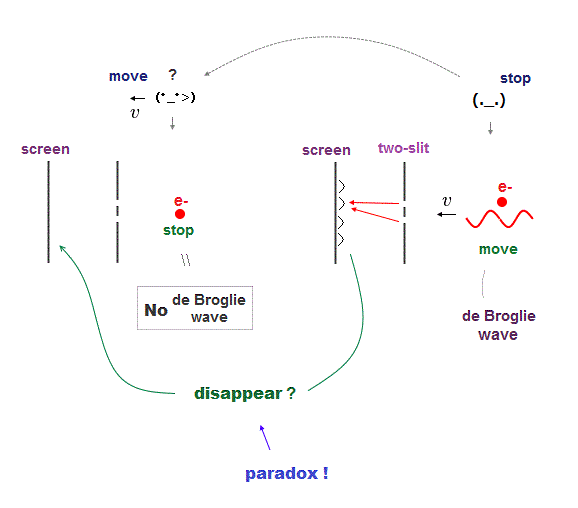When observers at rest and moving at v watch the same electron, they see the different interference patterns on the same screen ?

Seen from an observer at rest watching the same electron, the interference of de Broglie wave is recorded on the screen.

But seen from a moving observer, the electron appears to stop, so neither de Brolgie wave nor its interference of the electron can be seen !

This de Broglie wave paradox clearly shows Einstein famous relation is false.  de Broglie relation of the electron disobeys Lorentz contraction.

## An electron in quantum field theory is nonsense.

### [ Symbol "c" represents an electron ? ]

(Fig.11)  Quantum field theory cannot describe a concrete electron.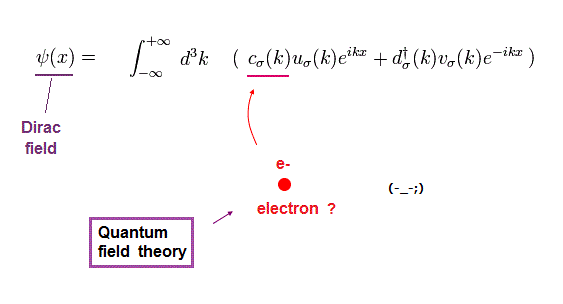All quantum field theories are based on relativistic Dirac equation.
How is each electron described in this quantum field theory ?

The problem is these quantum field theories have No ability to describe concrete figure and motion of each particle.

All it can show is an abstract and simple symbol "c" as an electron.
The detailed derivation is this.

## Electron = meaningless math symbol ?

### [ Quantum field theory cannot describe a true electron ! ]

(Fig.12)  It can do only two simple actions: create and vanish !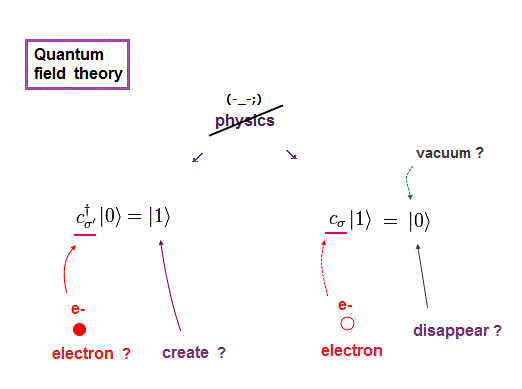Any quantum field theories cannot describe actual electrons.
It only shows a single letter (= "c ") as an electron.

Using this single letter, all quantum field theory can do is two actions: create and annihilate each electron !

When you see an electron ( here "a" ) and a photon, you will find the quantum field theory is valueless and useless for us.

## The present particle physics lacks reality.

### [ All particles by quantum field theory are unsubstantial. ]

(Fig.13)  Dirac field describes all electron, quark, neutrino ?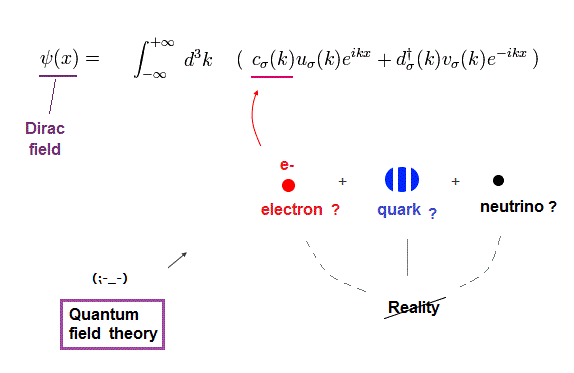Relativistic Dirac equation is supposed to describe "fermion".
"Fermion" includes electron, quark and neutrino.

It means all these particles are desctibed as a "single letter" like electron.  So quantum field theory cannot express each particle as an actual object.

Unless quantum field theory can explain actual, concrete state of each particle, it is completely useless and meaningless for us, forever.

## How does an electron emit a photon ?

### [ Electron and photon in quantum field theory are nonsense. ]

(Fig.14)  Emitted photon must always be "virtual", not real.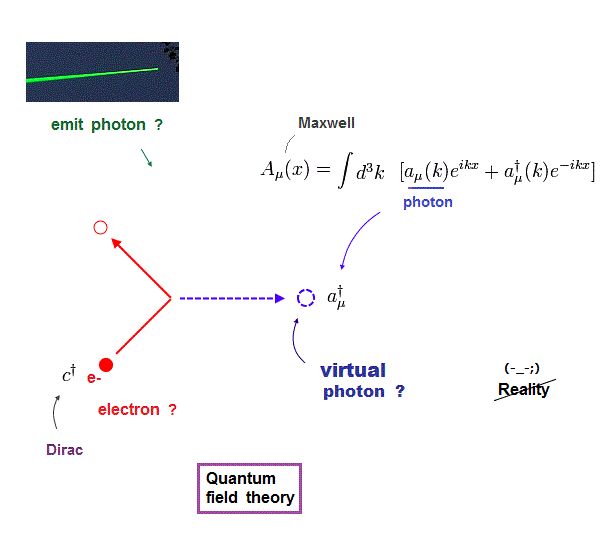It is said that quantum field theory (= QED ) can describe how each electron emits a photon (= light ).

But in fact, this quantum field theory can show nothing useful for these electron and photon.

An electron (= c ) and photon (= a ) are just abstract nonphysical symbols, so this emission process by quantum field theory is meaningless.

Furthermore, emitted photon in quantum field theory must be always " virtual" particle, which disobeys Einstein relation from the beginning.

So all relativistic quantum field theory is self-contradictory, of course, of No value for our daily lives.

## Virtual photon is unreal.

### [ All reactions need unreal virtual particles in quantum field theory. ]

(Fig.15)  Photoelectric effect and Coulomb by quantum field theory ?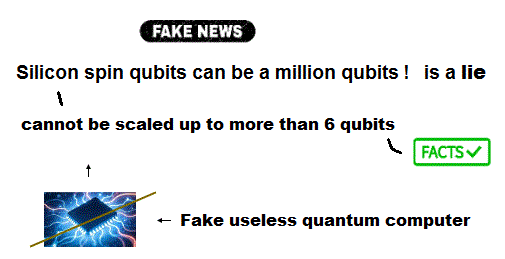In quantum field theory, all reactions including photoelectric effect must be described using abstract Feynman diagram.

The problem is all these processes in quantum field theory are always accompanied by unreal virtual particles.  See Coulomb interaction.

Why are they called "virtual" particle instead of "real" particle ?

For example, the mass squared of virtual photon can be negative violating Einstein relativity ( this p.5 ).

So photoelectric effect and Coulomb interaction by quantum field theory have nothing to do with actual phenomena, and useless for us.

## Pauli exclusion principle ?

### [ Pauli exclusion by quantum field theory is nonsense. ]

(Fig.16)  Pauli exclusion principle = anticommutation of two symbols ?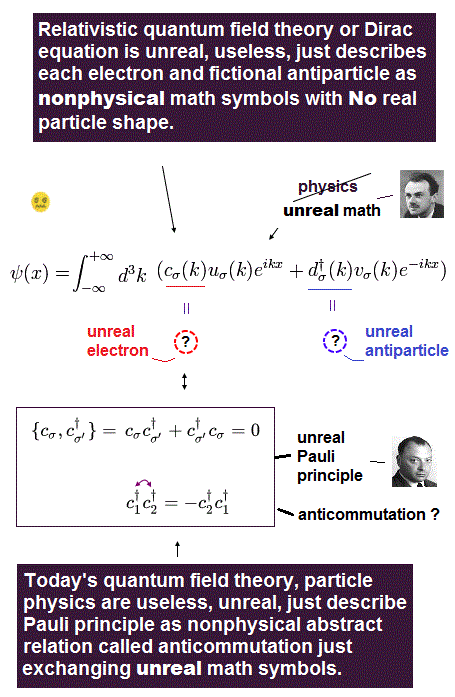Pauli exclusion principle claims two electrons cannot be in the same kind of state in orbital and spin, though spin is too weak to cause this.

It is said relativistic quantum field theory can explain why this Pauli exclusion principle occurs.  But it is a big lie.

All quantum field theory can show is anticommutaton of two electrons' symbols.  That's all.  No more detailed is mentioned.

If two electrons are in the same state ( c1 = c2 ), these c must be zero, when exchanging them reverses the whole sign (= anticommutation ).

So c1 and c2 must be different states from each other.  No more detailed mechanism in Pauli principle is mentioned in quantum field theory !

Quantum field theory does NOT try to clarify further mechanism in Pauli exclusion than this meaningless exchange relation.

## Quantum field theory is unreal.

### [ Quantum field theory describes nothing about real world. ]

(Fig.17)  Virtual particle, Higgs, 10 dimensional string are unreal.The conclusion is that quantum field theory based on Einstein relativity describes nothing about actual phenomena.

It just shows meaningless math symbol as each particle.
This is the reason all Higgs and string theory are out of touch with reality.

## QED is meaningless.

### [ QED artificially removes infinity to get finite value. ]

(Fig.18)  Feynman said QED is hocus-pocus.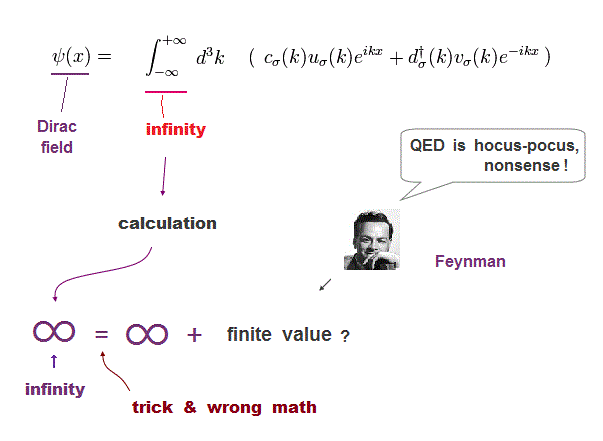Though you may often hear quantum electrodynamics (= QED ) is one of most successful theories, it is a big lie.

The problem is the calculation results of QED always diverge to infinity !  So they started to artificially remove a part of infinity by renormalization.

Infinity can be divided into other infinity and any arbitrary finite value.
If we remove this excessive infinity, we can get any finite value as we like.

This artificial trick is everything.  QED is NOT a successful theory at all.

Even founder, Feynman said "QED is hocus-pocus, and mathematically not consistent".

## Conclusion: the present physics is nonsense.

### [ The present physics is unreal, useless, waste of time. ]

(Fig.19)  Quantum field theory is Not physics !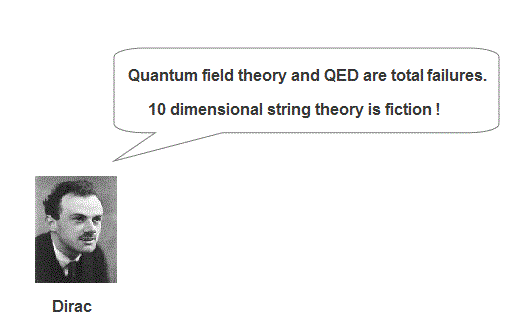The conclusion is that the present physics including quantum field theory cannot be called "physics".

It is filled with meaningless and mathematically inconsistent ideas.

Even Dirac said "it's a stop-gap procedure, there must be fundamental change as from Bohr's orbit to quantum mechanics".

Feynman, Dirac, nobody accepted fantasy 10 dimensional string theory.  The root of all evils is quantum mechanics which is just false.

## Where does our basic science stop ?

### [ Atomic force microscope sees each atom !  Next ... ? ]

(Fig.20)  If we can see each single atom, what can we do next ?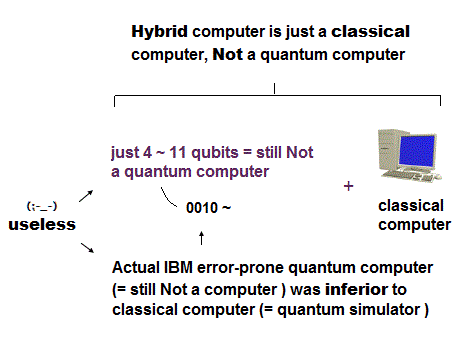Using atomic force microscope (= AFM ), we can observe each atom directly.  AFM can get informations by "trouching" and "feeling" the surface of atoms.

These informations of each atom includes various useful things such as electrical forces, potential and conductivity inside a single atom.

Then if we utilize this powerful AFM, we can know the concrete electron's behavior inside each atom ?

Unfortunately, the unrealistic quantum mechanics prevents us from doing it !

## Quantum mechanics stops our science.

### [ Density functional theory (= DFT ) is meaningless. ]

(Fig.21)  DFT cannot predict any electron's behavior.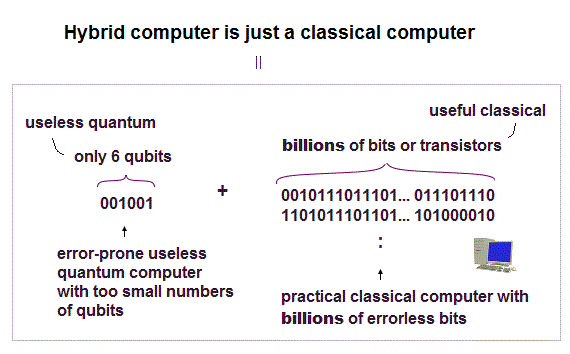For example, this news say, they could first get images of each molecule using atomic force microscope.

But when we see their paper ( Fig.3 ), you find they depend on density functional theory (= DFT ) as electron's model.

The problem is DFT just chooses artificial electron's functions as we like, so DFT has NO ability to predict any values and electron's behavior !

Other cases using atomic force microscope also depend on useless DFT model.  See this p.13.

Quantum mechanics can neither solve nor handle multi-electron atoms, because it discard two basic preconditions.

We must determine the more precise electronic motion model step by step, comparing experiments (= AFM data ) and the consistent model's prediction, giving up false quantum mechanics.

And when we try to manipulate each electron's behavior using electromagnetic wave, QED based on unreal virtual photon is an obstacle.

## Density functional theory ( DFT ) is useless.

### [ DFT can freely choose functional fitting experimental result. ]

(Fig.22)  Electron interaction term is freely chosen. ← useless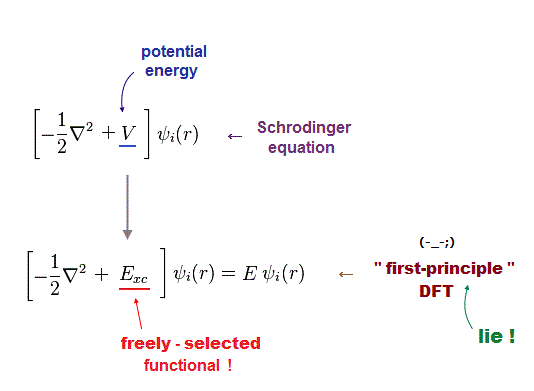In larger atoms in condended matter physics, density functional theory (= DFT ) is the only computing method even in atomic force microsopy.

It is often said this DFT is successful "ab-initio" method ( this p.3 ).
"Ab-initio" means first-principle which can predict values without empirical parameter ?

Unfortunately this DFT has No ability to predict any values, so useless.
Like this, DFT just chooses some convenient functional out of infinie choices.

In DFT, "exchange correlation functional" means interelectronic repulsions.  This functional is unknown, can be freely determined ( this p.2 ).

So DFT can be considered a semi-empirical method, different from media-hype ( this p.23 )2016/3/4 updated. Feel free to link to this site.